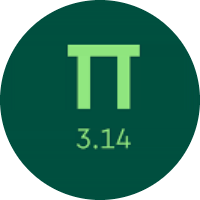# Understanding the Basics of Exponents

In this quiz, you will find mostly each type of problems related to the exponents like, powers of ten, multiplication of exponents with whole numbers, multiplication with decimal numbers, division of exponents, and evaluating exponents.

Start Quiz

Write the expression using an exponent.5^3

5^4

5^5

Evaluate.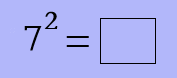49

7

14

Find the missing term.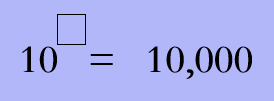3

5

4

Find the missing power.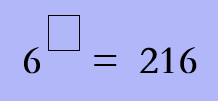4

5

6

Evaluate.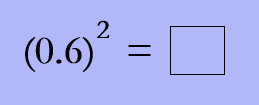36

3.6

0.36

Evaluate.32

0.0032

0.00032

Evaluate.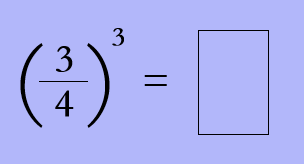27/16

9/32

27/64

Evaluate.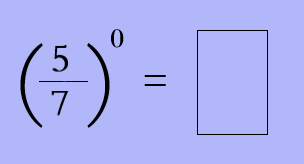0

5/7

1

Match the following.

Find the missing term.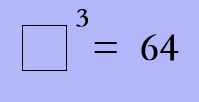4

8

5

Quiz/Test Summary
Title: Understanding the Basics of Exponents
Questions: 10
Contributed by: